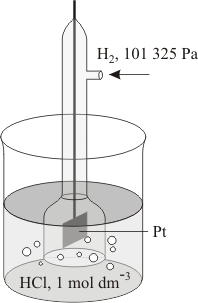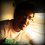# Physical Chemistry - Electrochemistry - 2Before reading this post, please read the first post here : Physical Chemistry - Electrochemistry - 1

STANDARD HYDROGEN ELECTRODE
A standard hydrogen electrode is prepared by dipping a Platinum strip in $1M HCl$ around which $H_2$ gas at $1$ atm pressure is bubbled in an inverted test tube. At $25^oC$, its standard reduction potential is defined to be zero.

STANDARD ELECTRODE POTENTIALS
As discussed in the previous post, the potential of any electrode is the potential difference between it and the electrolyte surrounding the electrode.
However, this potential depends upon the nature of the metal, concentration of the metallic ions in solution and the temperature of the solution.

Hence, again, we have to define a standard.
When the ions are at unit activity and the temperature is $25^oC$ ($298K$), the potential difference is called a Standard Electrode Potential ($E_o$).

Now,
the potential of a single electrode cannot be determined, but the potential difference between two electrodes can be accurately measured.
By convention, the electrode potential of S.H.E has been assigned a value of zero Volts.

POSITIVE(+) AND NEGATIVE(-) POTENTIALS
$\bullet$ If, on combining an electrode $A$ with the S.H.E, a reduction process (gain of electrons) occurs at the electrode, the potential of the electrode is called as reduction potential.
It is given a "+" sign as reduction potential or a "-" sign as oxidation potential.

$\bullet$ On the other hand, if, on combining an electrode $B$ with the S.H.E, an oxidation process (loss of electrons) occurs at $B$, the electrode potential (half-cell potential) is called as the oxidation potential.
It is given a "+" sign as oxidation potential or a "-" sign as reduction potential.

Thus, in short,
at Standard conditions,
Oxidation Potential for any half cell $=$ $-$(Reduction Potential)
Reduction Potential for any half cell $=$ $-$(Oxidation Potential)

In the next post, we shall discuss, the experimentally determined Electrochemical Series, and how it is used to determine the EMF of any cell.

Read the next post here : Physical Chemistry - Electrochemistry - 3Note by Anish Puthuraya
7 years, 3 months ago

This discussion board is a place to discuss our Daily Challenges and the math and science related to those challenges. Explanations are more than just a solution — they should explain the steps and thinking strategies that you used to obtain the solution. Comments should further the discussion of math and science.

When posting on Brilliant:

• Use the emojis to react to an explanation, whether you're congratulating a job well done , or just really confused .
• Ask specific questions about the challenge or the steps in somebody's explanation. Well-posed questions can add a lot to the discussion, but posting "I don't understand!" doesn't help anyone.
• Try to contribute something new to the discussion, whether it is an extension, generalization or other idea related to the challenge.

MarkdownAppears as
*italics* or _italics_ italics
**bold** or __bold__ bold
- bulleted- list
• bulleted
• list
1. numbered2. list
1. numbered
2. list
Note: you must add a full line of space before and after lists for them to show up correctly
paragraph 1paragraph 2

paragraph 1

paragraph 2

[example link](https://brilliant.org)example link
> This is a quote
This is a quote
    # I indented these lines
# 4 spaces, and now they show
# up as a code block.

print "hello world"
# I indented these lines
# 4 spaces, and now they show
# up as a code block.

print "hello world"
MathAppears as
Remember to wrap math in $$ ... $$ or $ ... $ to ensure proper formatting.
2 \times 3 $2 \times 3$
2^{34} $2^{34}$
a_{i-1} $a_{i-1}$
\frac{2}{3} $\frac{2}{3}$
\sqrt{2} $\sqrt{2}$
\sum_{i=1}^3 $\sum_{i=1}^3$
\sin \theta $\sin \theta$
\boxed{123} $\boxed{123}$

Sort by:

Why they use Hydrogen electrode as a standard? I thought gases is harder to handle than solid?

- 7 years, 3 months ago

It is generally done to form a good standard basis. This kind of stuff is done a lot, especially on linear algebra and it makes it somewhat "elegant". So this is something that we kinda agreed upon. To make a reference electrode because as this note says, we can't determine the potential of a single electrode... And really, it just forms a sort of like a baseline originating historically. Like "building" electrochemistry from scratch. I guess, think of it like 360$^\circ$ is one circle, why 360? Because of something people agreed upon years before (Babylonians had base 60 and ~360 days per year). x'D Hopefully, that's satisfying. I probably might've even interpreted your question incorrectly, but anyways, that's all I can say. ^^

- 7 years, 3 months ago

Well said.

- 7 years, 3 months ago

Well, Hydrogen is the first element, so I guess it is fair to choose it as a standard. I cant think of any other reason. If you don't choose Hydrogen as standard, what else would you choose? I dont know.

- 7 years, 3 months ago